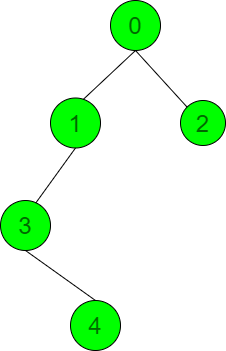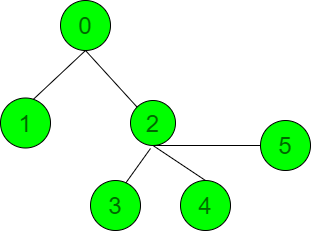# Maximum sum of distances of a node to every other node

• Difficulty Level : Hard
• Last Updated : 06 Oct, 2021

Given an undirected, connected tree with N nodes valued from 0 to N – 1 and an array edges[] represents the edges between two nodes, the task is to find the maximum sum of distances of a node to every other node in the tree.

Examples:

Attention reader! Don’t stop learning now. Get hold of all the important DSA concepts with the DSA Self Paced Course at a student-friendly price and become industry ready.  To complete your preparation from learning a language to DS Algo and many more,  please refer Complete Interview Preparation Course.

In case you wish to attend live classes with experts, please refer DSA Live Classes for Working Professionals and Competitive Programming Live for Students.

Input: N = 5, edges = { {0, 2}, {1, 3}, {0, 1}, {3, 4} }Output: 10
Explanation:
Considering the node 2 as the sources node, the distances of all other nodes from node 2 are: 1(node 0), 2(node 1), 3(node 3), 4(node 4). Therefore, the sum of distances is 1 + 2 + 3 + 4 = 10.

Input: N = 6, edges[][] = {{0, 1}, {0, 2}, {2, 3}, {2, 4}, {2, 5}}Output: 12

Naive Approach: The simplest approach to solve the given problem is to perform the Depth First Search Traversal from every node and find the sum of distance every other node from the current source node. After checking from all the nodes as the source node print the maximum sum among all the sum of values obtained.

Below is the implementation of the above approach:

## C++

 `// C++ program for the above approach`   `#include ` `using` `namespace` `std;`   `// Function to perform DFS and find the` `// distance from a node to every other` `// node` `void` `dfs(``int` `s, vector > g,` `         ``int` `p, ``int` `d, ``int``& ans)` `{` `    ``for` `(``int` `i : g[s]) {`   `        ``// If i is not equal to` `        ``// parent p` `        ``if` `(i != p) {` `            ``ans += d;` `            ``dfs(i, g, s, d + 1, ans);` `        ``}` `    ``}` `}`   `// Function to find the maximum sum of` `// distance from a node to every other` `// node` `void` `maxPotentialDistance(` `    ``int` `N, vector >& edges)` `{` `    ``int` `ans = 0;`   `    ``// Construct the graph` `    ``vector > g(N, vector<``int``>());`   `    ``for` `(``auto``& it : edges) {` `        ``g[it].push_back(it);` `        ``g[it].push_back(it);` `    ``}`   `    ``// Find the sum of distances from` `    ``// every node` `    ``for` `(``int` `i = 0; i < N; i++) {`   `        ``// Stores the maximum sum of` `        ``// distance considering the` `        ``// current node as source node` `        ``int` `a = 0;`   `        ``// Perform DFS Traversal to` `        ``// find the sum of distances` `        ``dfs(i, g, -1, 1, a);`   `        ``// Update the maximum sum` `        ``ans = max(ans, a);` `    ``}`   `    ``// Print the maximum sum` `    ``cout << ans;` `}`   `// Driver Code` `int` `main()` `{` `    ``int` `N = 6;` `    ``vector > edges = {` `        ``{ 0, 1 }, { 0, 2 }, { 2, 3 }, { 2, 4 }, { 2, 5 }` `    ``};`   `    ``maxPotentialDistance(N, edges);`   `    ``return` `0;` `}`

## Python3

 `# python program for the above approach`   `pd_0 ``=` `0`   `# Function to perform DFS and find the` `# distance from a node to every other` `# node`     `def` `dfs(s, g, p, d, count):` `    ``global` `pd_0` `    ``for` `i ``in` `g[s]:`   `                ``# If i is not equal to` `                ``# parent p` `        ``if` `(i !``=` `p):` `            ``pd_0 ``+``=` `d`   `            ``# Perform the DFS Traversal` `            ``dfs(i, g, s, d ``+` `1``, count)`   `            ``# Update the count of` `            ``# nodes` `            ``count[s] ``=` `count[s] ``+` `count[i]`     `# Function to find the distances from` `# every other node using distance from` `# node 0` `def` `dfs2(s, g, p, pd_all, n, count):`   `    ``for` `i ``in` `g[s]:`   `                ``# If i is not equal to the` `                ``# parent p` `        ``if` `(i !``=` `p):` `            ``pd_all[i] ``=` `pd_all[s] ``-` `count[i] ``+` `n ``-` `count[i]` `            ``dfs2(i, g, s, pd_all, n, count)`     `# Function to find the maximum sum of` `# distance from a node to every other` `# node` `def` `maxPotentialDistance(N, edges):` `    ``global` `pd_0` `    ``ans ``=` `0`   `    ``# Construct the graph` `    ``g ``=` `[[] ``for` `_ ``in` `range``(N)]`   `    ``for` `it ``in` `edges:` `        ``g[it[``0``]].append(it[``1``])` `        ``g[it[``1``]].append(it[``0``])`   `        ``# Stores the number of nodes in` `        ``# each subtree`   `    ``count ``=` `[``1` `for` `_ ``in` `range``(N)]`   `    ``# Find the sum of distances from` `    ``# node 0 and count the number of` `    ``# nodes in each subtree`   `    ``dfs(``0``, g, ``-``1``, ``1``, count)`   `    ``# Stores distances from each node` `    ``pd_all ``=` `[``0` `for` `_ ``in` `range``(N)]` `    ``pd_all[``0``] ``=` `pd_0`   `    ``# Find the distances from each` `    ``# node using distance from node 0`   `    ``dfs2(``0``, g, ``-``1``, pd_all, N, count)`   `    ``# Find the result`   `    ``for` `i ``in` `pd_all:` `        ``ans ``=` `max``(ans, i)`   `        ``# Print the result` `    ``print``(ans)`     `# Driver Code` `if` `__name__ ``=``=` `"__main__"``:`   `    ``N ``=` `6` `    ``edges ``=` `[` `            ``[``0``, ``1``], [``0``, ``2``], [``2``, ``3``], [``2``, ``4``], [``2``, ``5``]` `    ``]` `    ``maxPotentialDistance(N, edges)`   `    ``# This code is contributed by rakeshsahni`

Output:

`12`

Time Complexity: O(N2)
Auxiliary Space: O(N)

Efficient Approach: The above approach can also be optimized by observing that there is a relation between the sum of distances of the nodes to every other node. Let’s take node x. If y is its child, then it is observed that the sum of distances of y and x are related as;

distance of y = distance x – number of nodes in subtree y + nodes that are not in the subtree y

The required distances of all nodes can be calculated by calculating the sum of distances from one node and knowing the number of nodes in each subtree. Follow the steps below to solve the problem:

• Define a function dfs(int s, vector<vector<int> > graph, int p, int d, int &ans, vector<int>& count) and perform the following steps:
• Iterate over the range [0, graph[s]] using the variable i and if i is not equal to p, then increase the value of ans by d and call the function dfs(i, graph, s, d + 1, ans, count) to explore other nodes and set the value of count[s] as (count[s] + count[i]).
• Define a function dfs2(int s, vector<vector<int> > graph, int p, vector<int> &pd_all, int N, vector<int>& count) and perform the following steps:
• Iterate over the range [0, graph[s]] using the variable i and if i is not equal to p, then set the value of pd_all[i] as (pd_all[s] – count[i] + n – count[i]) and call the function dfs(i, graph, s, pd_all, N, count) to find answer for other nodes.
• Initialize the variable, say ans that stores the result.
• Construct an Adjacency List, graph[] from the given edges[][].
• Initialize the vector count[] of size N to keep track of the count of nodes in the subtree of a given node.
• Initialize the variable pd_0 as 0 to store the sum of distances from node 0 to every other node in the tree.
• Call the function dfs(s, graph, p, d, ans, count) to find the required distance from node 0 to every other node and store the count of number of nodes in a subtree.
• Initialize the vector pd_all[] of size N to store the distances from every other node.
• Set the value of pd_all as pd_0.
• Call the function dfs2(s, graph, p, pd_all, N, count) to find the required distances from every other node.
• Iterate over the range [0, N] using the variable i and update the value of ans as the maximum of ans or pd_all[i].
• After performing the above steps, print the value of ans as the answer.

Below is the implementation of the above approach:

## C++

 `// C++ program for the above approach` `#include ` `using` `namespace` `std;`   `// Function to perform DFS and find the` `// distance from a node to every other` `// node` `void` `dfs(``int` `s, vector > g,` `         ``int` `p, ``int` `d, ``int``& ans,` `         ``vector<``int``>& count)` `{` `    ``for` `(``int` `i : g[s]) {`   `        ``// If i is not equal to` `        ``// parent p` `        ``if` `(i != p) {` `            ``ans += d;`   `            ``// Perform the DFS Traversal` `            ``dfs(i, g, s, d + 1,` `                ``ans, count);`   `            ``// Update the count of` `            ``// nodes` `            ``count[s] = count[s] + count[i];` `        ``}` `    ``}` `}`   `// Function to find the distances from` `// every other node using distance from` `// node 0` `void` `dfs2(``int` `s, vector > g,` `          ``int` `p, vector<``int``>& pd_all,` `          ``int` `n, vector<``int``> count)` `{` `    ``for` `(``int` `i : g[s]) {`   `        ``// If i is not equal to the` `        ``// parent p` `        ``if` `(i != p) {` `            ``pd_all[i] = pd_all[s]` `                        ``- count[i]` `                        ``+ n - count[i];` `            ``dfs2(i, g, s, pd_all,` `                 ``n, count);` `        ``}` `    ``}` `}`   `// Function to find the maximum sum of` `// distance from a node to every other` `// node` `void` `maxPotentialDistance(` `    ``int` `N, vector >& edges)` `{` `    ``int` `ans = 0;`   `    ``// Construct the graph` `    ``vector > g(N, vector<``int``>());`   `    ``for` `(``auto``& it : edges) {` `        ``g[it].push_back(it);` `        ``g[it].push_back(it);` `    ``}`   `    ``// Stores the number of nodes in` `    ``// each subtree` `    ``vector<``int``> count(N, 1);` `    ``int` `pd_0 = 0;`   `    ``// Find the sum of distances from` `    ``// node 0 and count the number of` `    ``// nodes in each subtree` `    ``dfs(0, g, -1, 1, pd_0, count);`   `    ``// Stores distances from each node` `    ``vector<``int``> pd_all(N, 0);` `    ``pd_all = pd_0;`   `    ``// Find the distances from each` `    ``// node using distance from node 0` `    ``dfs2(0, g, -1, pd_all, N, count);`   `    ``// Find the result` `    ``for` `(``int` `i : pd_all)` `        ``ans = max(ans, i);`   `    ``// Print the result` `    ``cout << ans;` `}`   `// Driver Code` `int` `main()` `{` `    ``int` `N = 6;` `    ``vector > edges = {` `        ``{ 0, 1 }, { 0, 2 }, { 2, 3 }, { 2, 4 }, { 2, 5 }` `    ``};` `    ``maxPotentialDistance(N, edges);`   `    ``return` `0;` `}`

Output:

`12`

Time Complexity: O(N)
Auxiliary Space: O(N)

My Personal Notes arrow_drop_up
Recommended Articles
Page :